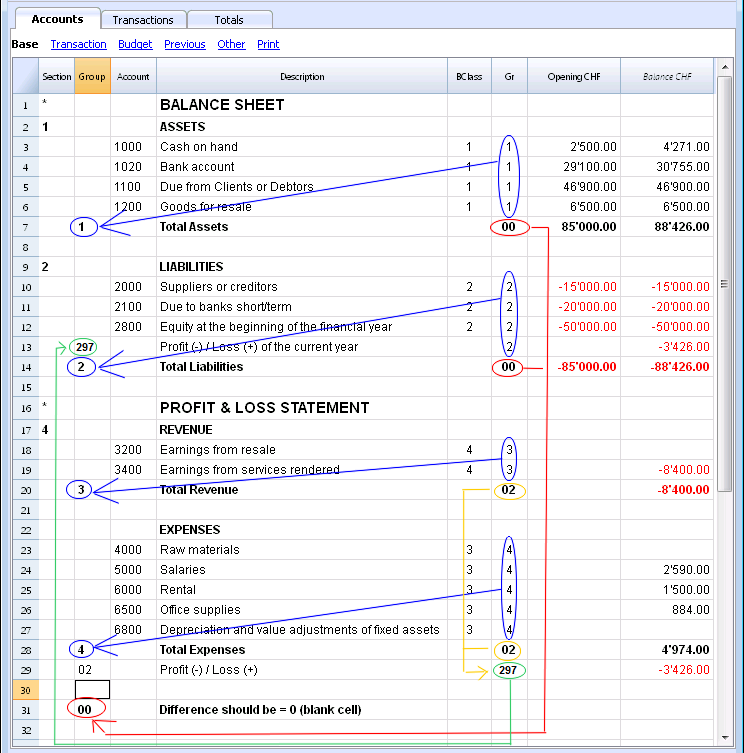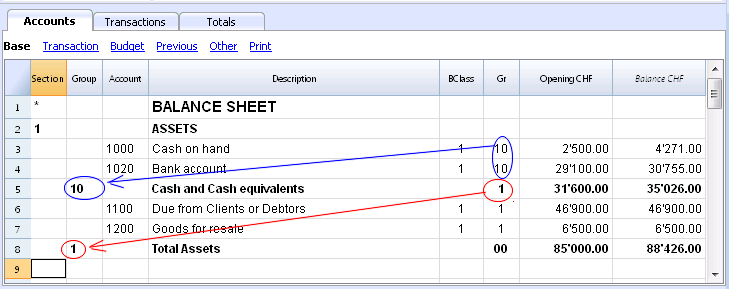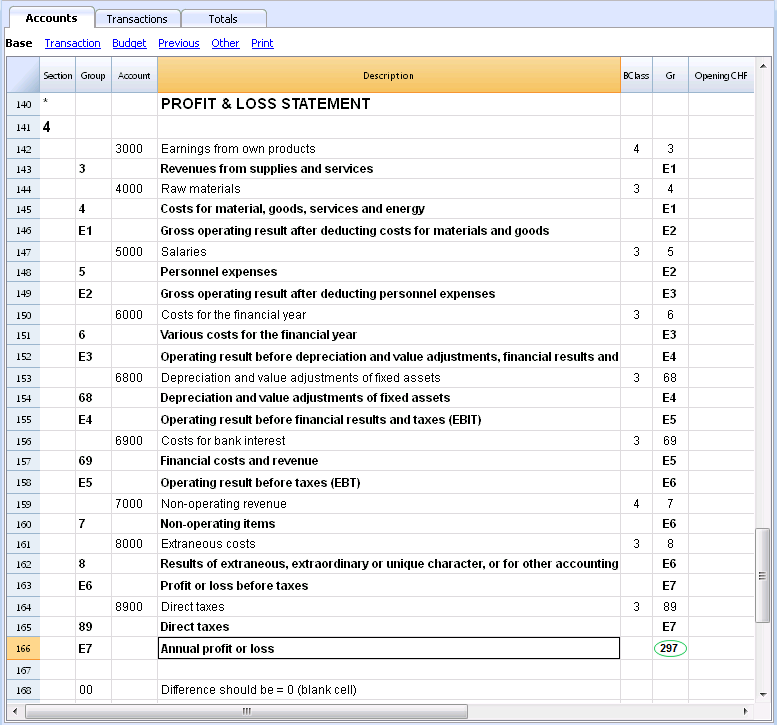# Groups

## The grouping and totaling system

The Banana grouping and totaling system is based on the following columns:

• Group (TOTAL row)
When, in a row, a group identifier is being entered, the row becomes a TOTAL row.
In this row the amounts of the Gr column, that contain the same identifier, are being totaled.
When a group is present, there cannot be an account. .
• Gr (Adds this row into the TOTAL row)
The number here must be one of the numbers defined in the Group column.

With this system, an account can be totaled in a group row, the group in another group and so on... in order to create different totaling levels.
This totaling system is very powerful and flexible, but it takes some "getting used" to it, in order to be able use it well.

## Examples of the main groupes in the Double-entry accounting

Every Chart of accounts uses its own totaling system. Hereunder the main groupes of the Double-entry accounting are being explained.

In the Double-entry accounting, the total of the Debit balances (positive) together with the Credit balances (negative) have to result in 0 (zero).

• The 00 is the row where all the amounts are being added together.
It is the "Summa summarum" of all the Debit & Credit balances.
In case the Balances columns do not amount to zero, it means there is a Debit/Credit difference and thus most likely a mistake. In these cases it is useful to proceed with a checking of the accounting (see next point).
In the Debit and Credit columns of the Transactions view (Accounts table), there are only positive amounts, so also in the row of the 00 group, there will be the total of the transctions not equal to zero.

The calculation sequence to arrive at 00 is therefore as follows:

• The 1000 account -> group 1 (Total Assets)-> Group 00
• The 2000 account -> group 2 (Total Liabilities) -> Group 00
• The 3000 account -> group 3 (Total Revenue) -> Group 02 (result Profit & Loss Statement)  ->  Group 297 (Profit/Loss of the current year in the Balance Sheet) -> Group 2 (Total Liabilities) -> Group 00.
• The 4000 account -> group 4 (Total Expenses) -> Group 02 (result Profit & Loss Statement)  ->  Group 297 (Profit/Loss of the current year in the Balance Sheet) -> Group 2 (Total Liabilities) -> Group 00.

### The result of profit & loss statement is added in equity

As you can see in the example, the Group 02 (Profit /Loss from Profit & Loss statement) is totalized in the 297 liabilities group (current year result).
With this group organization, we have several advantages:

• In current year operating result is displayed in the balance sheet
• The Total Liabilities will match the Total Assets (provided that there is no accounting error).With this system it is easy to add totaling levels.
When we want to create a subgroup for the Cash & Cash equivalents accounts:

• Enter an empty row after the bank account
• Enter the value 10 into the Group column
• Enter the value 1 into the Gr column
• Indicate the Gr 10 in the accounts 1000 et 1020
• The sequence for the calculation becomes:
The 1000 account -> Group 10 (Cash & Cash equivalents) -> Group 1 (Total Assets) -> Group 00.In case you want to insert another subgroup, "Current Assets", proceed in the same way.

• Add an empty row above the row of the Total Assets.
• In the new row:
• Indicate the number 11 in the Group column
• Indicate the number 1 in the Gr column
• In the Clients and Goods for resale (inventory) rows, indicate the Gr 11.

### Deleting subgroups

In case the Chart of accounts shows subgroups that are no longer needed or not wanted, these can be deleted. Just delete the row of the subgroup and modify the Gr of every account that was part of that subgroup.

### Checking of the structure

Once the Chart of accounts has been set up, execute the Check accounting command of the Account1 menu. In case there are errors, the program issues a warning.

### Infinite loop error

This warning appears when a Group is being totaled in a Group of a lower level, reason for which an infinite error loop is being created.
There would be an infinite loop when, in the preceding example, the Assets Group (1) would be totaled in Group 10.
The program, after having calculated the Group 1, would total the amount in Group 10, which in turn would total the amount in Group 1, and then again in 10 without ending.

## Profit & Loss Statement with Gross Profit

It is also possible to use a Profit & Loss Statement that starts with the total Business result and that subtracts the costs.
Hereunder the example of the Swiss PME Chart of Accounts is shown.## Extended use of the totaling system

The totaling system is very flexible:

• In one Group, it is possible to add Groups as well as Accounts
This can be seen in the earlier shown example where the Group Cash & cash equivalents (10) and the accounts (1100 and 1300) are being totaled in the Assets
• You can use whatever kind of numbering
• You can create up to a hundred totaling levels
• The Total row can be after the rows
• The Total row can be before the rows
• The Total row can be completely elsewhere, in a separate position
This is being used to create registers (for example, a client register)
• The accounts and the Groups can be totaled in one single other Group.
It is not possible to total one and the same account at the same time in two groups.
• This system is also being used to total the Cost centers and the Segments
Pay attention though to not use different groups for normal accounts and for cost centers, because the resulting amounts will not be correct.

### Totaling of the Amount columns

The calculation procedure totals the amount columns

• The amount columns defined by the system are being totaled.
• The amount columns added by the user are being totaled.

### The sequence of the calculation

The programme calculates the totals in the following manner:

• Sets the values of the Amount columns of the Groups rows on zero
• Totals the amounts of the Account rows into the Group rows (first calculation level)
• Adds the balance of the Group rows into the Group(s) of a superior level
Repeats the operation until all the levels have been calculated.

### Related Documents

#### This documentation is outdated

Search in the latest documentation

The feature you are looking for is missing? Try the new version! Banana Accounting+